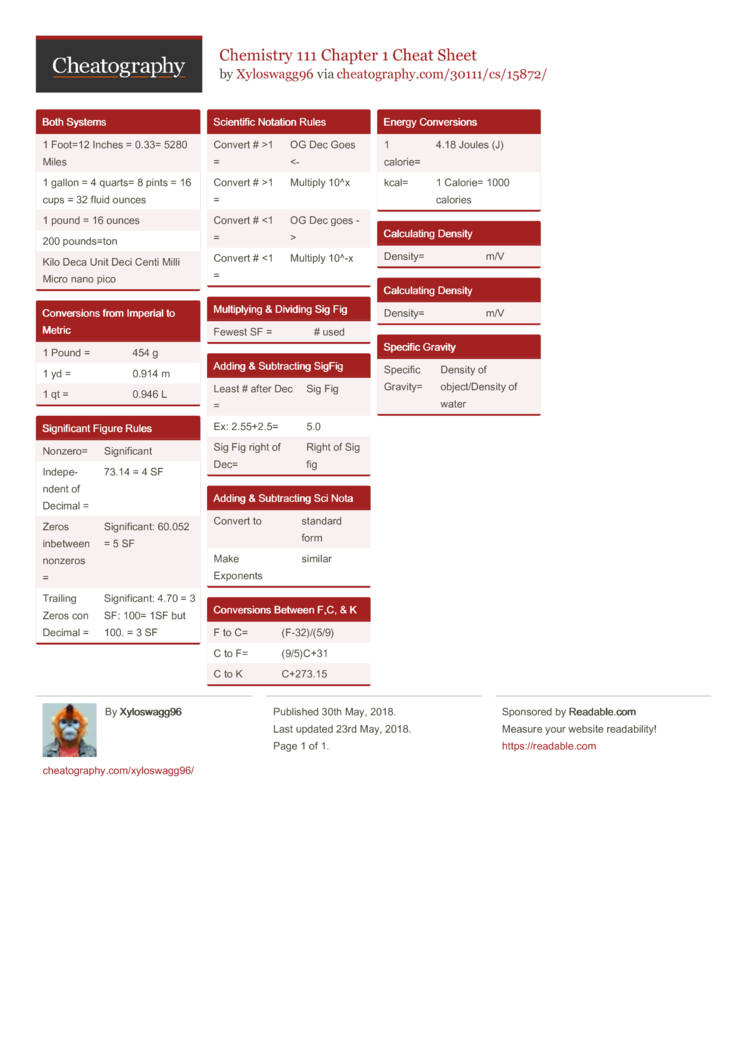# Chemistry 111 Chapter 1 Cheat Sheet by Xyloswagg96

A lot of conversions

### Both Systems

 1 Foot=12 Inches = 0.33= 5280 Miles 1 gallon = 4 quarts= 8 pints = 16 cups = 32 fluid ounces 1 pound = 16 ounces 200 pounds=ton Kilo Deca Unit Deci Centi Milli Micro nano pico

### Conver­sions from Imperial to Metric

 1 Pound = 454 g 1 yd = 0.914 m 1 qt = 0.946 L

### Signif­icant Figure Rules

 Nonzero= Signif­icant Indepe­ndent of Decimal = 73.14 = 4 SF Zeros inbetween nonzeros = Signif­icant: 60.052 = 5 SF Trailing Zeros con Decimal = Signif­icant: 4.70 = 3 SF: 100= 1SF but 100. = 3 SF

### Scientific Notation Rules

 Convert # >1 = OG Dec Goes <- Convert # >1 = Multiply 10^x Convert # <1 = OG Dec goes -> Convert # <1 = Multiply 10^-x

### Multip­lying & Dividing Sig Fig

 Fewest SF = # used

 Least # after Dec = Sig Fig Ex: 2.55+2.5= 5.0 Sig Fig right of Dec= Right of Sig fig

### Adding & Subtra­cting Sci Nota

 Convert to standard form Make Exponents similar

### Conver­sions Between F,C, & K

 F to C= (F-32)­/(5/9) C to F= (9/5)C+31 C to K C+273.15

### Energy Conver­sions

 1 calorie= 4.18 Joules (J) kcal= 1 Calorie= 1000 calories

### Calcul­ating Density

 Density= m/V

### Calcul­ating Density

 Density= m/V

### Specific Gravity

 Specific Gravity= Density of object­/De­nsity of water1 Page
//media.cheatography.com/storage/thumb/xyloswagg96_chemistry-111-chapter-1.750.jpg

PDF (recommended)